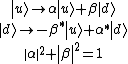# Unitary Symmetry

Also found in: Dictionary, Thesaurus, Medical, Acronyms.
Related to Unitary Symmetry: Unitary group

## Unitary symmetry

A type of symmetry law, an important example of which is flavor symmetry, one of the approximate internal symmetry laws obeyed by the strong interactions of elementary particles. According to the successful theory of strong interactions, quantum chromodynamics, flavor symmetry is the consequence of the fact that the so-called glue force (mediated by the SU3color gauge field) is the same between all the kinds (flavors) of quarks. If the quarks all had the same mass, they then would be dynamically equivalent constituents of hadrons, and hadrons would occur as degenerate multiplets of the group SUN, where N is the number of quark flavors. The lightest quarks (u and d) have similar masses, so the lightest hadrons, made of u and d quarks, do exhibit an SU2flavor symmetry known as i-spin invariance. The mass of the next heavier quark (s) is much larger than the masses of the u and d quarks, but much smaller than the masses of the yet heavier quarks (c, b, …); consequently the hadrons that contain no quarks heavier than the s quark clearly may be grouped into SU3flavor multiplets. See Color (quantum mechanics), Flavor, Hadron, Quantum chromodynamics, Quarks

An example of unitary symmetry is the approximate spin independence of the forces on electrons (as in an atom): There is a fundamental doublet, comprising the spin-up electron and the spin-down electron. Denoting these two states by &verbar;u〉 and &verbar;d〉, all physical properties (energy eigenvalues, charge density, and so on) are unchanged by the replacements shown in the equations below,where α and β are complex numbers. The group of all the transformations of two states that preserves their hermitean scalar products [〈u&verbar;d〉 = 0, 〈u&verbar;u〉 = 〈d&verbar;d〉 = 1] is known as the two-dimensional unitary group, U2; the transformations of the equations above form a subgroup SU2 which merely lacks the uninteresting transformations of the form &verbar;u〉 → ei&phiv;&verbar;u〉 and &verbar;d〉→ei&phiv;&verbar;d〉, that is, an equal change of phase of the two states.

The strong interactions are approximately invariant to an SU2 group; the fundamental doublet can be taken to be the nucleon, with the up and down states proton and neutron. This SU2 symmetry is known as charge independence, or, loosely, as i-spin conservation, the analog to the electron spin being known as i-spin I . See I-spin

When a sufficient number of strange particles had been observed, it was seen that they, together with the old nonstrange particles, were grouped into multiplets of particles with the same space-time quantum numbers (except for mass; the masses of the members are only similar, not equal). This suggested the existence of a yet larger symmetry; it has turned out that this symmetry is the group of all unitary transformations of a triplet of fundamental particles, U3, or SU3 if the uninteresting equal phase change of all particles is omitted. This symmetry is often loosely called unitary symmetry. See Strange particles

A striking difference in the manifestations of SU2 and SU3 is that whereas all possible multiplets of the former appear in nature, only those multiplets of the latter appear that can be regarded as compounds of the fundamental triplet in which the net number of component fundamental particles (number of particles minus number of antiparticles) is an integral multiple of 3. In particular, no particle that could be regarded as the fundamental triplet is found. Despite this nonappearance, it turns out that a great deal about the strongly interacting particles (hadrons) is at least qualitatively explained if they are regarded as physical compounds of a fundamental triplet of particles, to which the name quark has been given. The color theory (quantum chromodynamics) of strong interactions explains why single quarks are never observed.

According to the argument given above, hadrons have the approximate symmetry SUN, where N is the number of kinds of quarks, or flavors. Six flavors of quark are known; in addition to the quarks with the flavors up, down, and strange described above, three more quarks, charm, bottom, and top, have been found. See Charm, Elementary particle, J/psi particle, Upsilon particles

McGraw-Hill Concise Encyclopedia of Physics. © 2002 by The McGraw-Hill Companies, Inc.
The following article is from The Great Soviet Encyclopedia (1979). It might be outdated or ideologically biased.

## Unitary Symmetry

(or SU3 symmetry), an approximate symmetry of the strong interactions of elementary particles. Unitary symmetry reflects the existence of common properties in groups of hadrons and plays an important role in the classification of hadrons. It is a broader symmetry than isotopic invariance.

Unitary symmetry establishes the presence of intrinsic relations between particles that belong to different isotopic multiplets and that have a different strangeness. Particles with different values of isotopic spin and strangeness or hypercharge but with identical spin and intrinsic parity are combined into groups called supermultiplets. If unitary symmetry is to be strictly satisfied, the particles within a single supermultiplet should have the same masses; however, in reality, the masses differ quite strongly. The differences are explained by the existence of a moderately strong interaction that breaks unitary symmetry. The known hadrons form supermultiplets that consist of one, eight, and ten particles (seeSTRONG INTERACTION).

The Great Soviet Encyclopedia, 3rd Edition (1970-1979). © 2010 The Gale Group, Inc. All rights reserved.

## unitary symmetry

[′yü·nə‚ter·ē ′sim·ə·trē]
(particle physics)
An approximate internal symmetry law obeyed by the strong interactions of elementary particles; a system of particles has such a symmetry if all the particles can be described as compounds of a fundamental multiplet of particles, and if all physical properties of the system are unchanged by an arbitrary unitary transformation of this fundamental multiplet.
McGraw-Hill Dictionary of Scientific & Technical Terms, 6E, Copyright © 2003 by The McGraw-Hill Companies, Inc.
Site: Follow: Share:
Open / Close